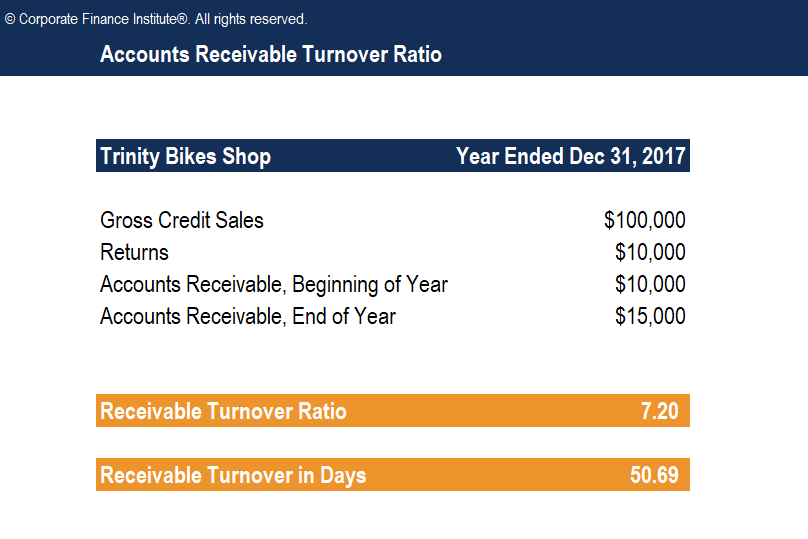# Accounts Receivable Turnover Ratio Template

## Accounts Receivable Turnover Ratio Template

This Accounts Receivable Turnover Ratio Template will show you how to calculate receivable turnover ratio and receivable turnover in days.

Below is the screenshot of the template:### What is the Accounts Receivable Turnover Ratio?

The accounts receivable turnover ratio, also known as the debtor’s turnover ratio, is an efficiency ratio that measures how efficiently a company is using its assets. The accounts receivable turnover ratio measures the number of times over a given period that a company collects its average accounts receivable.

### Accounts Receivable Turnover Ratio Formula

The accounts receivable turnover ratio formula is as follows:

Accounts Receivable Turnover Ratio = Net Credit Sales / Average Accounts Receivable

Where:

• Net credit sales are sales where the cash is collected at a later date. The formula for net credit sales is = Sales on credit – Sales returns – Sales allowances.
• Average accounts receivable is the sum of starting and ending accounts receivable over a time period (such as monthly or quarterly), divided by 2.

More Free Templates

For more resources, check out our business templates library to download numerous free Excel modeling, PowerPoint presentation and Word document templates.

• Excel Modeling Templates
• PowerPoint Presentation Templates
• Transaction Document Templates

### Financial Analyst Certification

Become a certified Financial Modeling and Valuation Analyst (FMVA)® by completing CFI’s online financial modeling classes and training program!